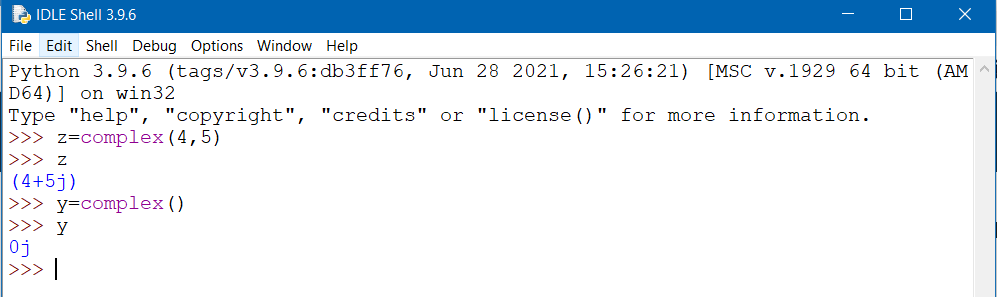### Python class numbers complex

The subclass complex numbers is employed to perform operations on numbers of complex type. The operations such as addition, subtraction, multiplication, division, retrieving imaginary and real part can be performed.
A complex number is defined as:
z=a+bj
where, a and b are real and complex part of the imaginary number. The type of the entity is known using keyword type(z).
type(z)
The code snippet when run in python IDLE is shown:
>>> z=2+4j
>>> type(z)
<class 'complex'>
>>>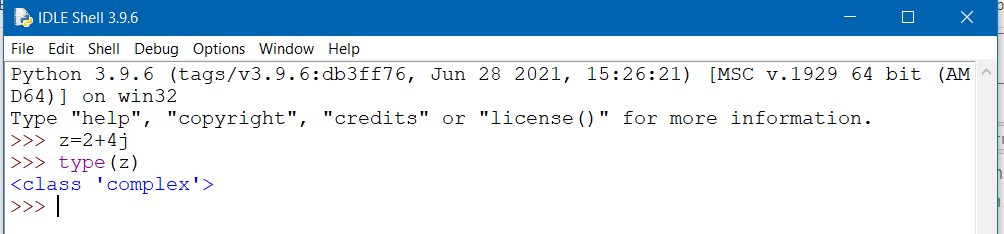Further, all calculations can be performed on complex numbers similar to natural numbers.
>>> z=2+4j
>>> type(z)
<class 'complex'>
>>> c=9+6j
>>> z+c
(11+10j)
>>> z-c
(-7-2j)
>>> z*c
(-6+48j)
>>> z/c
(0.3589743589743589+0.20512820512820515j)
>>> z**c
(927.6866288474685+94.93421503433717j)
>>>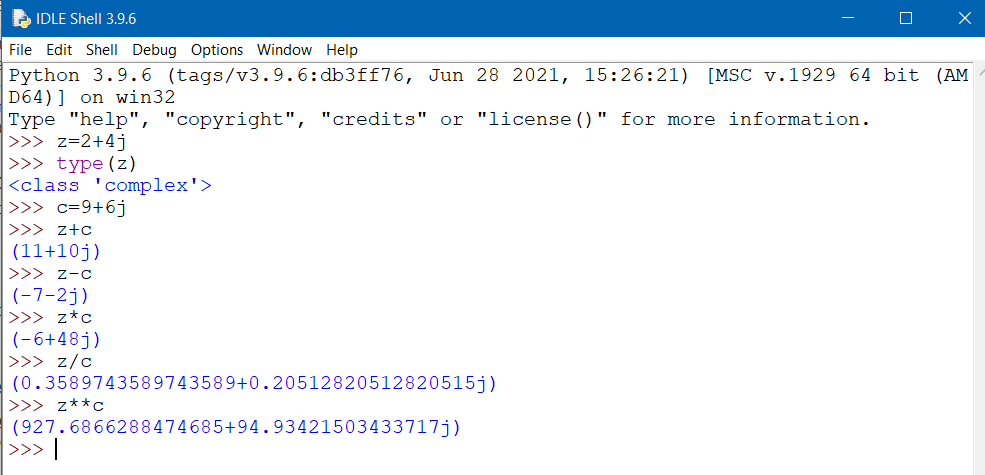In order to obtain complex conjugate of the complex number, conjugate() is used.
>>> z=3.4+9.89j
>>> z.conjugate()
(3.4-9.89j)
>>>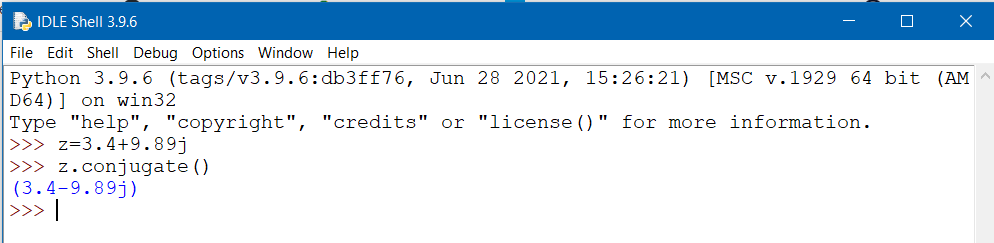Further, relational operators is applied to the complex numbers which returns TRUE or FALSE based upon the condition. The code snippet below depicts the equality of the two complex numbers z1 and z and returns true if the real and imaginary parts are equal.
>>> z=3.4+9.89j
>>> z.conjugate()
(3.4-9.89j)
>>> z1=9.89j+3.4
>>> z==z1
True
>>>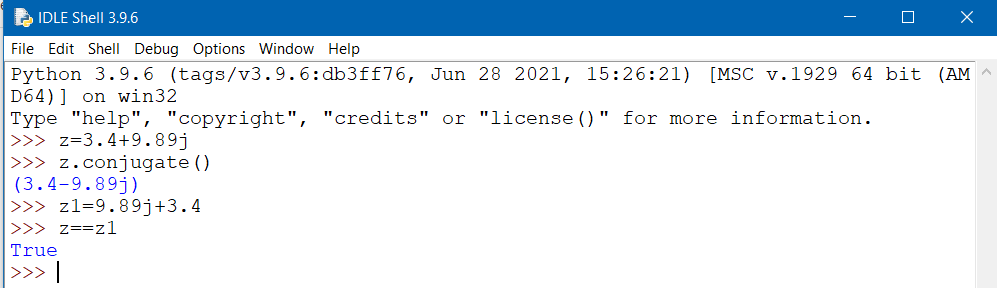Another relational operator, which checks if the two complex numbers are unequal and return TRUE if the numbers are unequal else returns FALSE, as depicted by the code snippet below.
>>> a=3+2j
>>> b=2j+3
>>> c=3j+2
>>> a!=b
False
>>> a!=c
True
>>>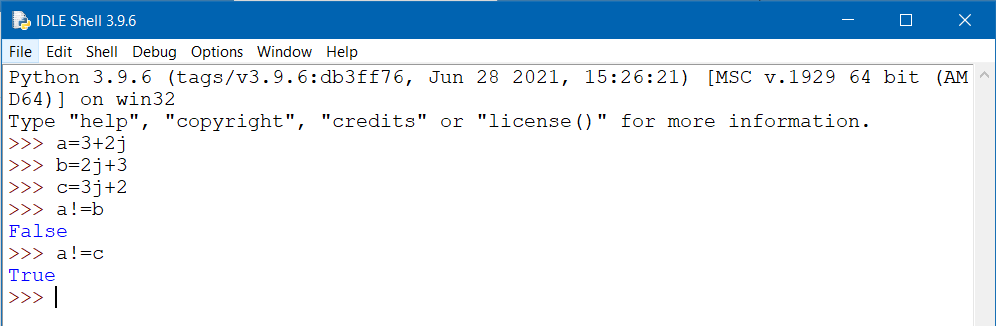The inbuilt complex() function can be used to define a complex number in the form complex(a,b) where a and b are real and imaginary part of the complex number. If no value is assigned to a and b, python takes default values of 0.
Depicted code snippet for the complex() function.
>>> z=complex(4,5)
>>> z
(4+5j)
>>> y=complex()
>>> y
0j
>>>